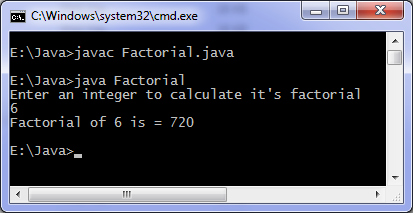# Java program to find factorial

Levels of difficulty: / perform operation:

This java program finds factorial of a number. Entered number is checked first if its negative then an error message is printed.

## Java Source Code

```import java.util.Scanner;

class Factorial
{
public static void main(String args[])
{
int n, c, fact = 1;

System.out.println("Enter an integer to calculate it's factorial");
Scanner in = new Scanner(System.in);

n = in.nextInt();

if ( n < 0 )
System.out.println("Number should be non-negative.");
else
{
for ( c = 1 ; c <= n ; c++ )
fact = fact*c;

System.out.println("Factorial of "+n+" is = "+fact);
}
}
}
```

### OutputYou can also find factorial using recursion, in the code fact is an integer variable so only factorial of small numbers will be correctly displayed or which fits in 4 bytes. For large numbers you can use long data type.